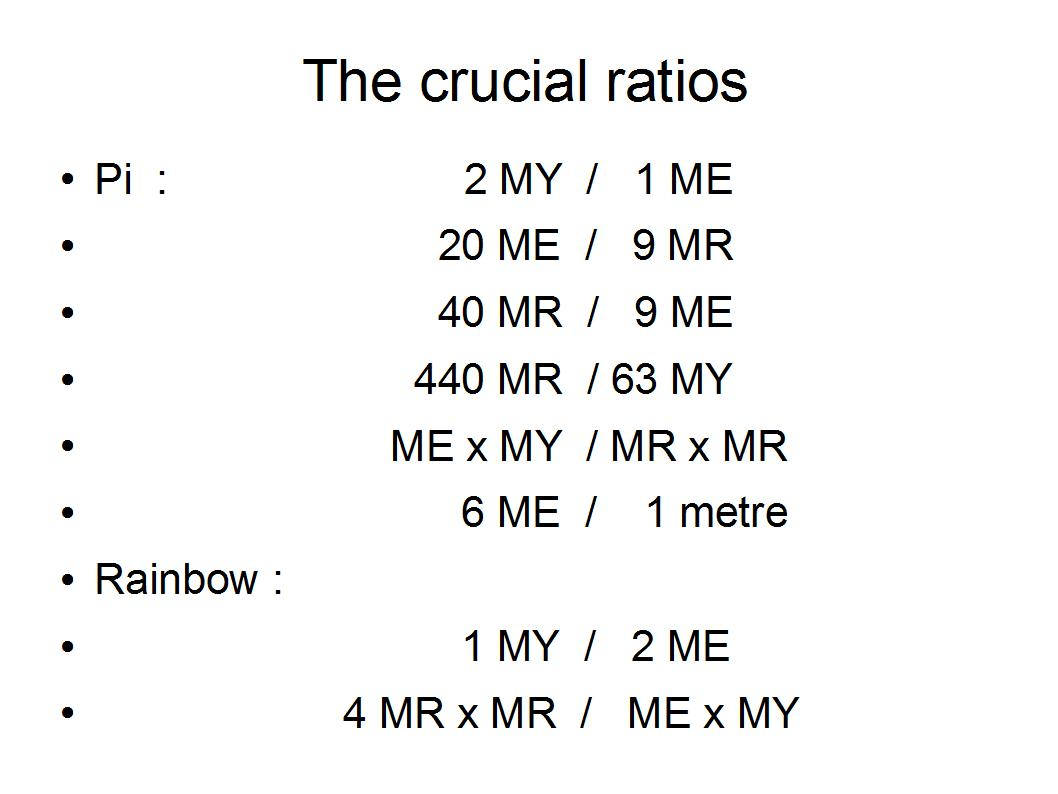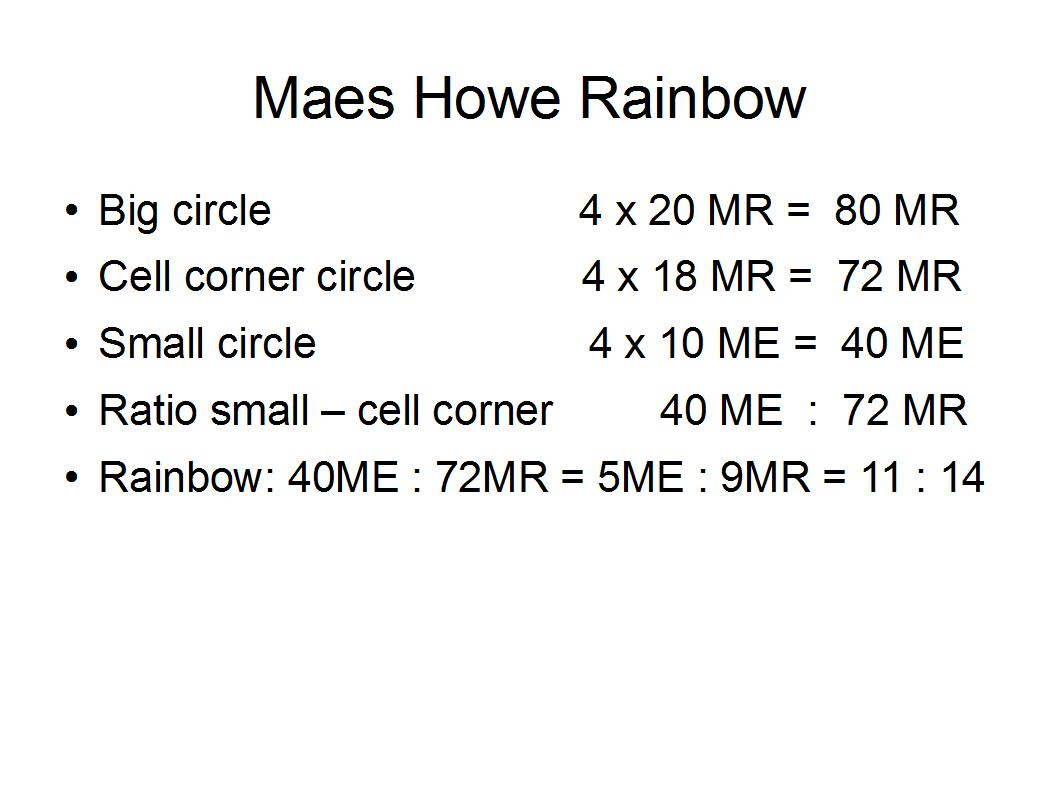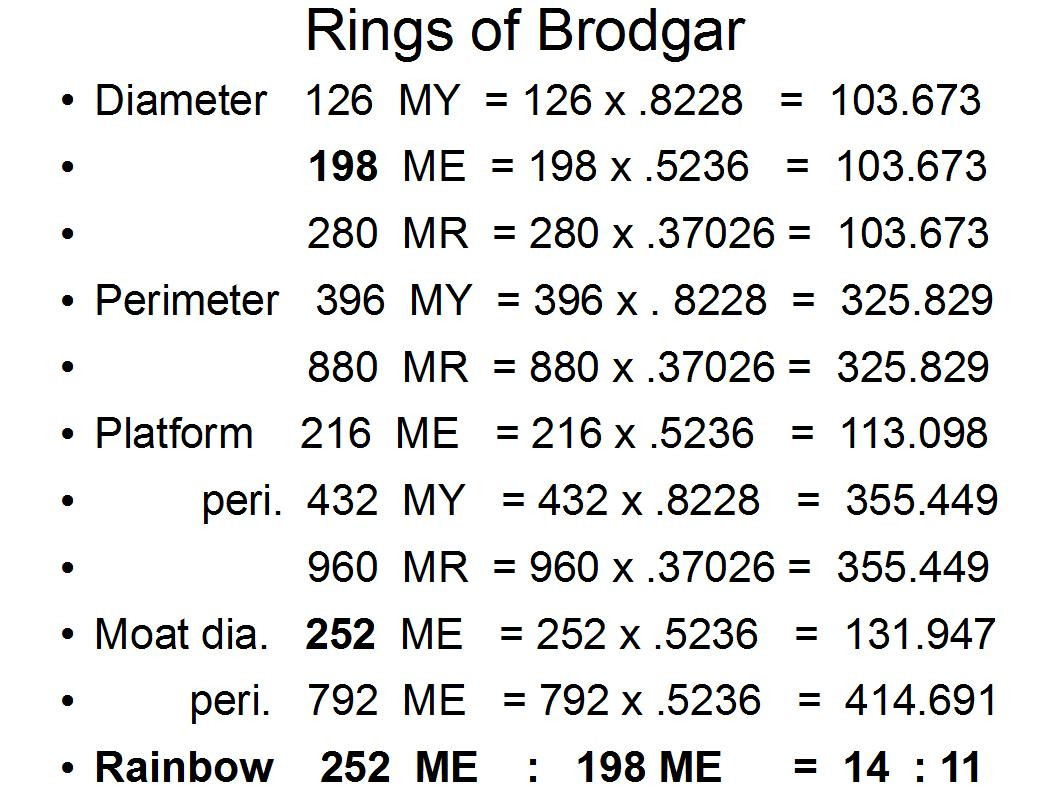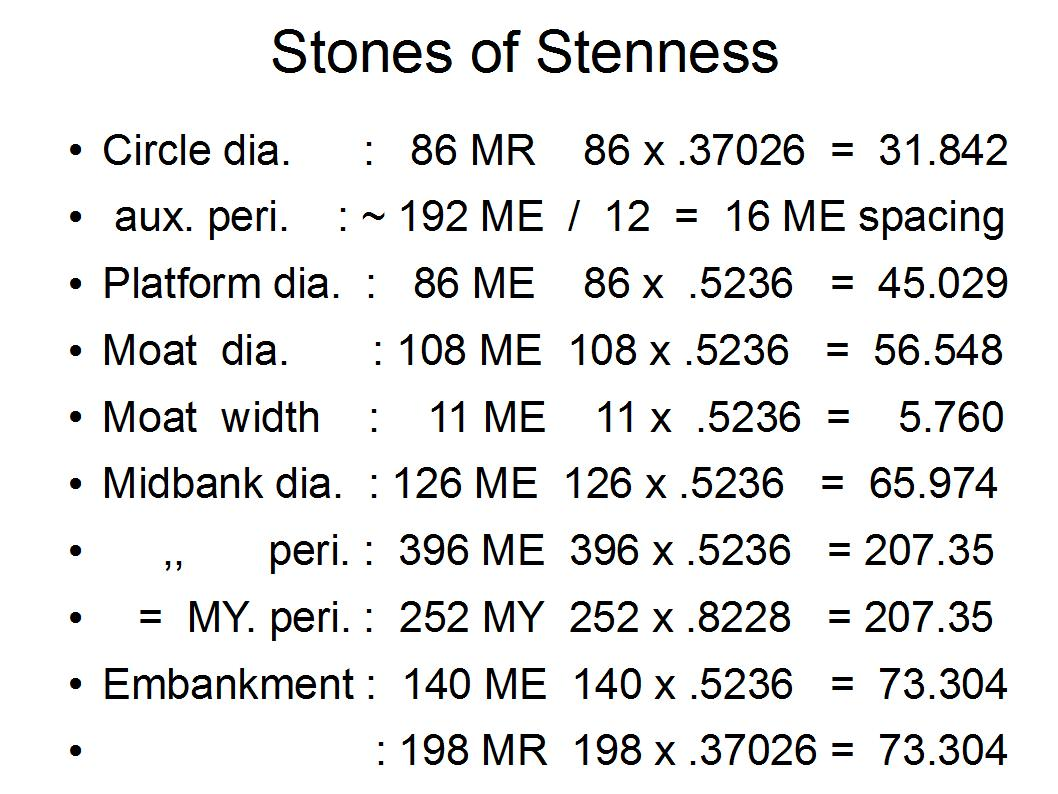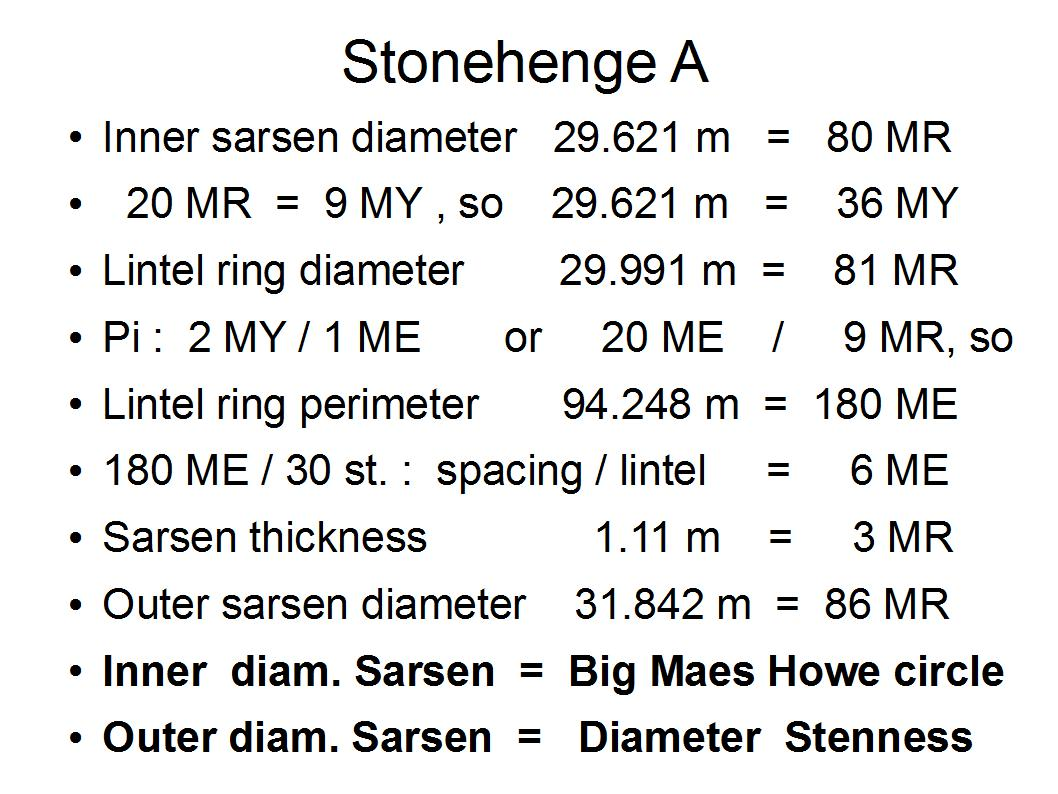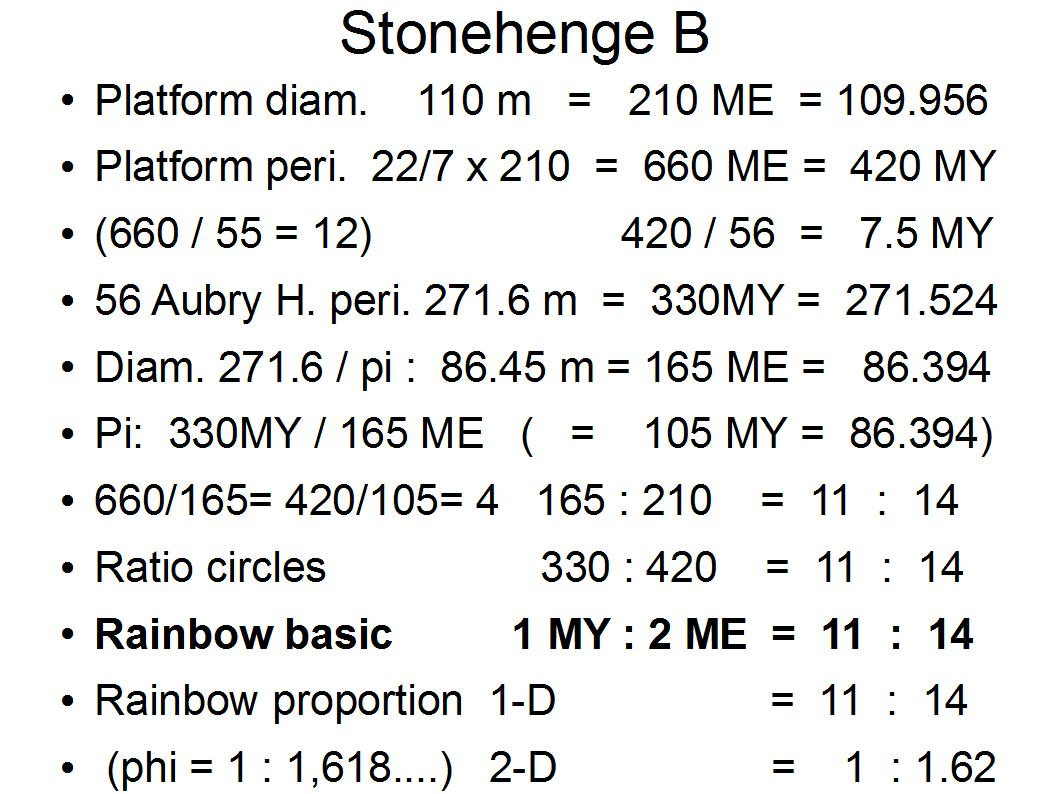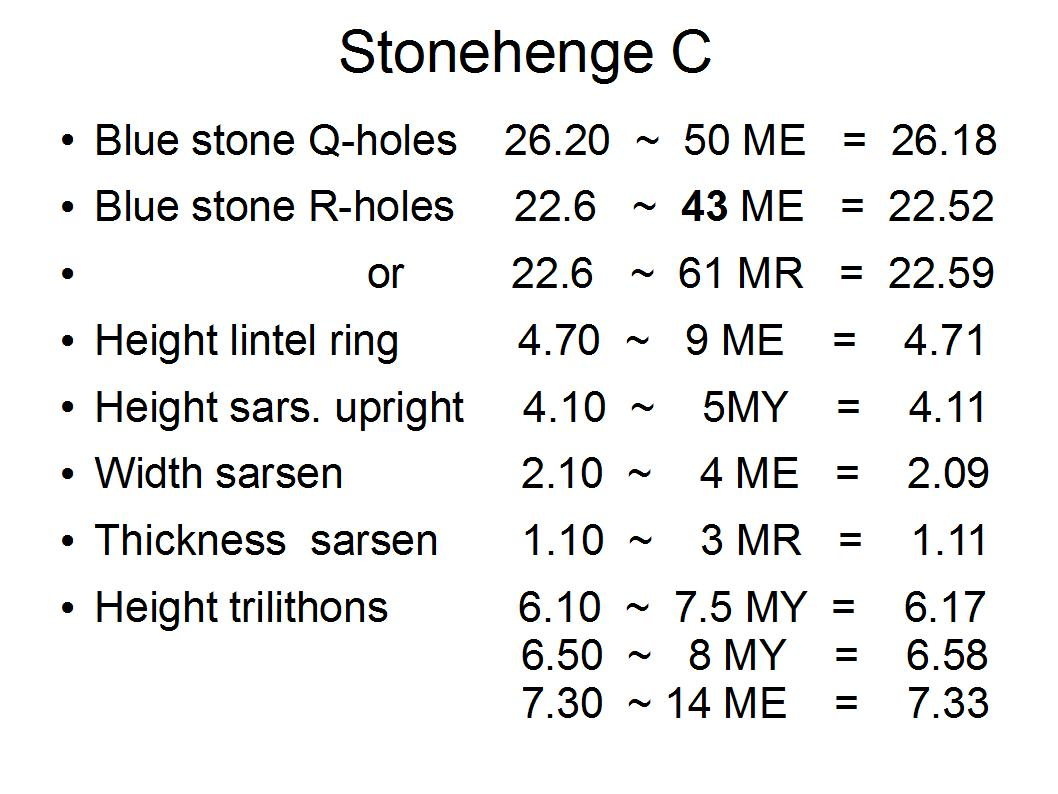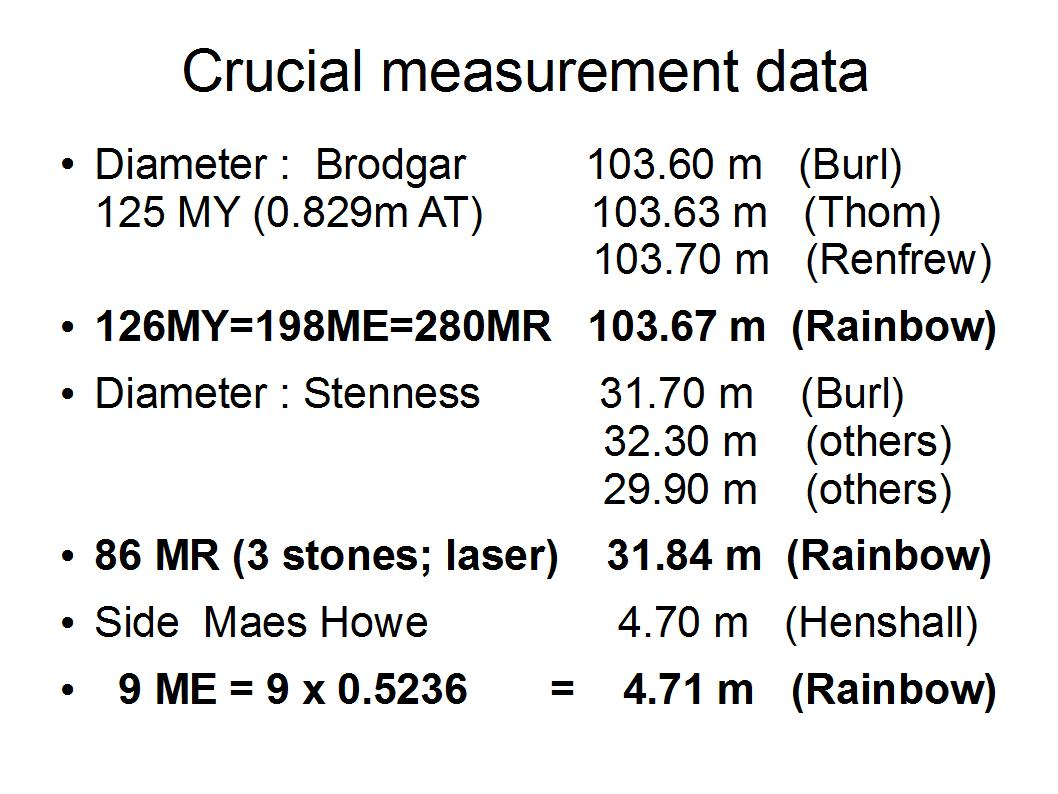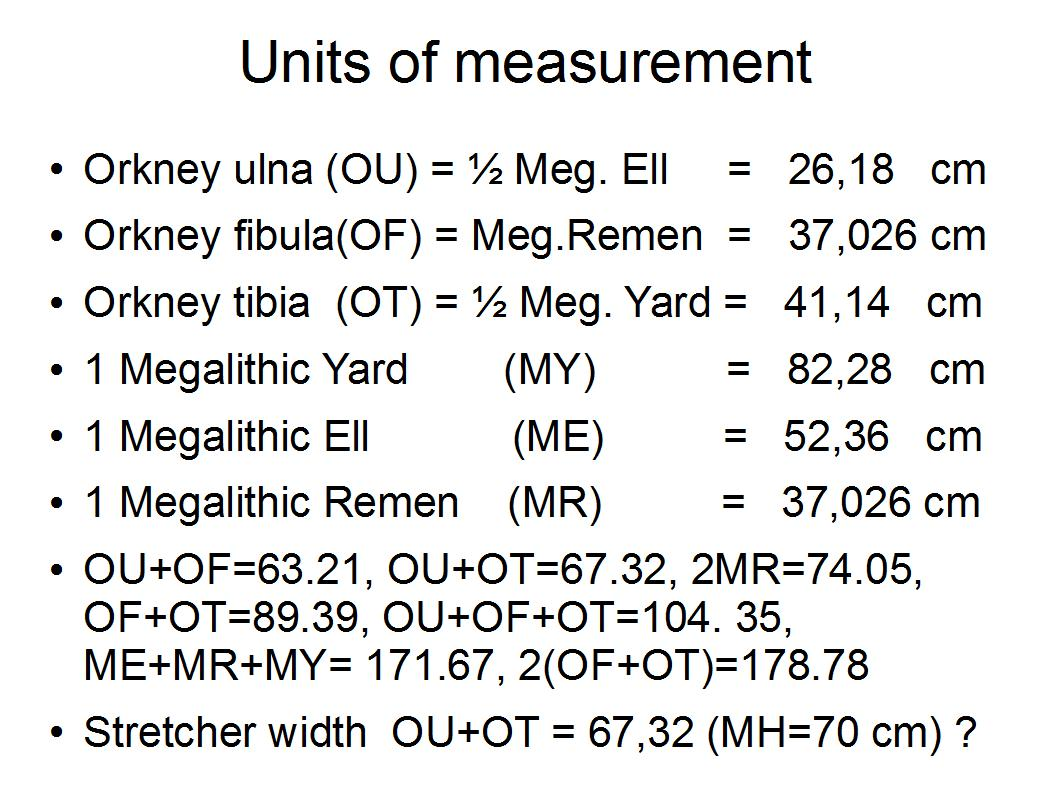## The Neolithic Numbers

Introduction

These are the bare data of major works of the British Isles, Maeshowe, Ring of Brodgar, Stones of Stenness and Stonehenge, all translated into the units of measurement as employed on this website. This is the system I have created on the basis of already known units which I have crafted in such a way that they have values which stand in a mathematical order. [differences of thousandths (like 0.009 etc.) and less are considered ‘exact’ matches throughout these pages, it is an integral aspect of the model of approximation]

The units are the following, respectively: Megalithic Ell (ME), -Remen (MR), -Yard (MY), -Foot (MF), these are all based on human bone lengths of the average Neolithic skeleton, the Ell on 2 male ulna (fore-arm), here called Orkney Ulna (OU), the Remen on 1 female fibula (calf) (OF), the Yard on 2 male tibia (shin) (OT), the Foot on 1 female humerus (upper-arm) (OH).

Ell (ME) = 52.360cm —– Ulna (OU) = 26.18cm
Remen (MR) = 37.025cm —– = Fibula (OF)
Yard (MY) = 82.280cm —– Tibia (OT) = 41.14cm
Foot (MF) = 29.620cm —– = Humerus (OH)

These values relate as follows:

11 ME = 7 MY
9 MY = 20 MR = 25 MF
99 ME = 140 MR

11 OU = 7 OT
9 OT = 10 OF
4 OF = 5 OH

the upper row works out as:

63 MY =  99 ME =  140 MR =  175 MF

We see that this is a fully integrated calculator, that it is in principle simple, but can be confusing because of the doubles and the halves and the ensuing ratios because for instance:

11 ME = 7 MY, but as a ratio it is 1 ME : 1 MY = 7 : 11, which looks like a mistake but is not, since

52.36 : 82.28 = 7 : 11 (52.36/7 = 7.48, this x 11 = 82.28)

So use a calculator when you think it is wrong, I have made mistakes in the past myself, but I think I’ve taken out most mistakes. If there are still mistakes I apologize for that, it does though not affect the consistency of the model as it stands. Remember it are all ‘approximations’ in terms of accepted mathematics

The practical explanation and applications of these numbers are given elsewhere (The Neolithic Numbers explained). This ordering is to show the recurrence of the same numbers and ratios in all the different buildings.